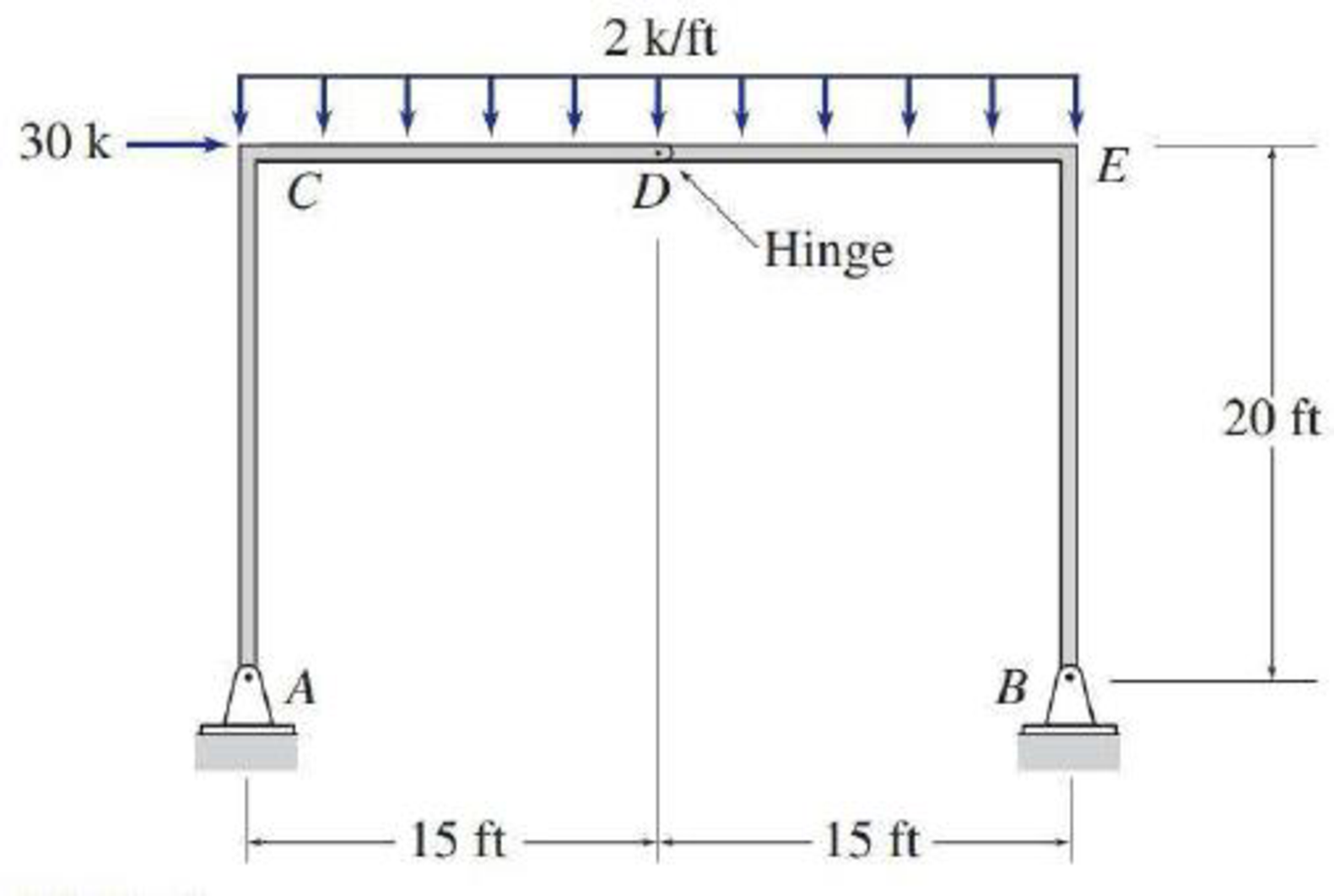# 5.57 through 5.71 Draw the shear, bending moment, and axial force diagrams and the qualitative deflected shape for the frame shown.

#### Solutions

Chapter
Section
Chapter 5, Problem 70P
Textbook Problem
298 views

## 5.57 through 5.71 Draw the shear, bending moment, and axial force diagrams and the qualitative deflected shape for the frame shown.To determine

Plot the shear diagram, bending moment diagram, axial force diagram, and the qualitative deflected shape of the frame.

### Explanation of Solution

Write the condition for static instability, determinacy and indeterminacy of plane frames as follows:

3m+r<3j+ecstaticallyunstableframe        (1)

3m+r=3j+ecstaticallydeterminateframe        (2)

3m+r>3j+ecstaticallyindeterminateframe        (3)

Here, number of members is m, number of external reactions is r, the number of joints is j, and the number of elastic hinges is ec.

Find the degree of static indeterminacy (i) using the equation;

i=(3m+r)(3j+ec)        (4)

Refer to the Figure in the question;

The number of members (m) is 3.

The number of external reactions (r) is 4.

The number of joints (j) is 4.

The number of elastic hinges ec is 1.

Substitute the values in Equation (2);

3(3)+4=3(4)+113=13staticallydeterminateframe

Show the free-body diagram of the entire frame as in Figure 1.

Refer Figure 1,

Consider entire frame.

+MA=0By(30)2(30)(302)30(20)=030By900600=0By=50k

Find the vertical reaction at point A by resolving the vertical component of forces.

+Fy=0Ay2(30)+By=0Ay60+50=0Ay=10k

Find the horizontal reaction at point A by resolving the horizontal component of forces.

+Fx=0Ax+30Bx=0        (1)

Consider the section DEB.

Find the horizontal reaction at point B by taking moment about the hinge at D.

+MDDEB=0By(15)2(15)(152)Bx(20)=050(15)225Bx(20)=0Bx=26.25k

Substitute 26.25 k for Bx in Equation (1).

Ax+3026.25=0Ax=3.75k

Show the free-body diagram of the members and joints of the entire frame as in Figure 2.

Consider point A:

Resolve the vertical component of forces.

+FY=010AYAC=0AYAC=10k

Resolve the horizontal component of forces.

+FX=0AXAC3.75=0AXAC=3.75k

Consider the member AC:

Resolve the vertical component of forces.

+FY=0AYACCYAC=010CYAC=0CYAC=10k

Resolve the horizontal component of forces.

+FX=0AXAC+CXAC=03.75+CXAC=0CXAC=3.75k

Take moment about the point C.

+MCAC=0MCACAXAC(20)=0MCAC3.75(20)=0MCAC=75k-ft

Consider the point C:

Resolve the vertical component of forces.

+FY=0CYACCYCE=010CYCE=0CYCE=10k

Resolve the horizontal component of forces

### Still sussing out bartleby?

Check out a sample textbook solution.

See a sample solution

#### The Solution to Your Study Problems

Bartleby provides explanations to thousands of textbook problems written by our experts, many with advanced degrees!

Get Started

Find more solutions based on key concepts
In Chapter 11, we explained the concept of windchill factors. We said that the heat transfer rates from your bo...

Engineering Fundamentals: An Introduction to Engineering (MindTap Course List)

Describe the hardware referred to by the terms, system unit and chassis.

Enhanced Discovering Computers 2017 (Shelly Cashman Series) (MindTap Course List)

Determine the axial force in member BC of the frame. Note the joint D is a sliding pin.

International Edition---engineering Mechanics: Statics, 4th Edition

How can a social networking site be used in a DSS?

Fundamentals of Information Systems

List three methods of applying cutting fluids.

Precision Machining Technology (MindTap Course List)

What causes most firewall failures?

Network+ Guide to Networks (MindTap Course List)

What is the purpose of the solid flux that covers the electrode?

Welding: Principles and Applications (MindTap Course List)

If your motherboard supports ECC DDR3 memory, can you substitute non-ECC DDR3 memory?

A+ Guide to Hardware (Standalone Book) (MindTap Course List)